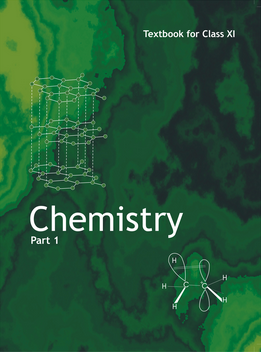# Basic concepts of Chemistry (Weightage 2%)   Share

### Topics from Basic concepts of Chemistry

• Chemical reaction (4 concepts)
• General introduction : Important and scope of chemistry (6 concepts)
• Atomic and molecular masses (3 concepts)
• Mole concept and molar masses (1 concepts)
• Percentage composition and & empirical and molecular formula (2 concepts)
• Stoichiometry and calculations based on stoichiometry (2 concepts)
• Matter and its nature (2 concepts)
• Dalton's atomic theory (4 concepts)
• Concept of atom, molecule, element and compund (5 concepts)
• Physical quantities and their measurements in chemistry (1 concepts)
• Precision and accuracy, significant figures, S.I. Units, dimensional analysis (9 concepts)
• Laws of chemical combination (4 concepts)
• Atomic and molecular masses, mole concept, molar mass (5 concepts)
• Percentage composition, Empirical and molecular formulae (2 concepts)
• Chemical equations and stiochiometry (6 concepts)

### Important Books for Basic concepts of Chemistry

•Exams
Articles
Questions